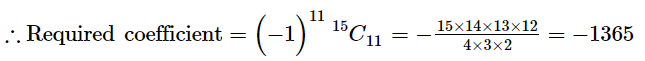# The coefficient of

Question:

The coefficient of $x^{-17}$ in the expansion of $\left(x^{4}-\frac{1}{x^{3}}\right)^{15}$ is

(a) 1365

(b) −1365

(c) 3003

(d) −3003

Solution:

(b) −1365

Suppose the $(r+1)$ th term in the given expansion contains the coefficient of $x^{-17}$.

Then, we have :

$T_{r+1}={ }^{15} C_{r}\left(x^{4}\right)^{15-r}\left(\frac{-1}{x^{3}}\right)^{r}$

$=(-1)^{r}{ }^{15} C_{r} x^{60-4 r-3 r}$

For this term to contain $x^{-17}$, we must have :

$60-7 r=-17$

$\Rightarrow 7 r=77$

$\Rightarrow r=11$